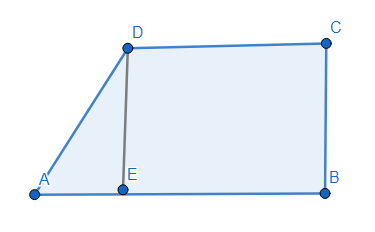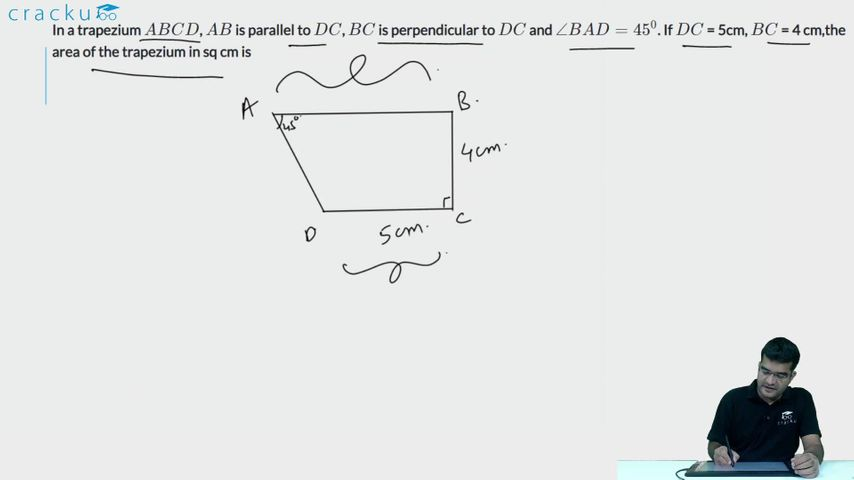Question 72

# In a trapezium $$ABCD$$, $$AB$$ is parallel to $$DC$$, $$BC$$ is perpendicular to $$DC$$ and $$\angle BAD=45^{0}$$. If $$DC$$ = 5cm, $$BC$$ = 4 cm,the area of the trapezium in sq cm is

SolutionGiven, BC = DE = 4

CD = BE = 5

In triangle ADE, EAD=45^{0}\tan\ 45\ =\ \frac{DE}{AE} => AE = 4

Area of trapezium = Area of rectangle BCDE + Area of triangle AED

= 20 + 8 = 28

### View Video Solution